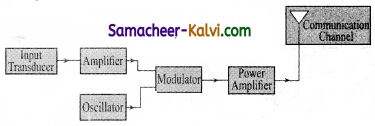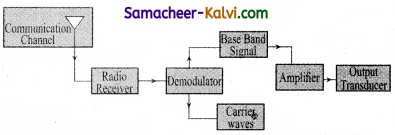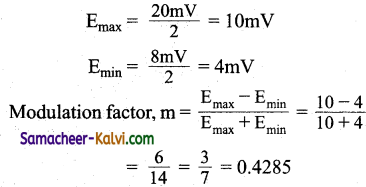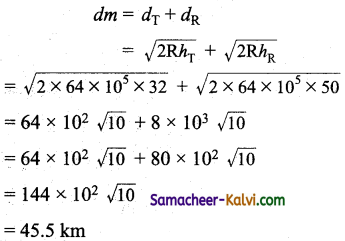## TN State Board 12th Physics Important Questions Chapter 10 Communication Systems

Question 1.
What are the different types of radio wave propagation?
The different types of radio wave propagation are:
(a) ground (surface) wave propagation.
(b) space wave propagation
(c) skywave (ionospheric propagation)

Question 2.
Name the types of communication systems according to the mode of the transmission.

• Analog communication system
• Digital communication system.

Question 3.
Define modulation.
Modulation is the process by which some characteristic, usually amplitude, frequency or phase angle of a high frequency earner wave is varied in accordance with the instantaneous value of the low frequency baseband signal input signal called modulation.Question 4.
Write the factors which justify the need of modulating a low frequency signal into high frequencies before transmission.
Modulation is needed,
(i) To transmit a low frequency signal to a distant place so that it may not die out in the way itself.
(ii) For protecting the waveform of the signal and
(iii) To keep the height of antenna small.

Question 5.
Whichismoreefficientmodeoftransmission. FM or AM?
FM transmission is more efficient because all the transmitted power is useful but in AM transmission most of the power goes waste in transmitting the carrier alone.

Question 6.
Why do we need a higher bandwidth for transmission of music compared to that for commercial telephonic communication?
Speech signals contain frequencies between 300 Hz to 3100 Hz. Such signals require a small bandwidth of 2800 Hz for telephonic communication. Audio signals have frequencies between 20 Hz to 20 kHz. So, the transmission of a good music requires a higher bandwidth of about 20 kHz.Question 7.
Give one example each of a ‘system’ that uses the (i) Sky wave, (ii) Space wave mode of propagation.
(i) Short broadcast services use sky wave propagation.
(ii) TV broadcast / microwaves link / satellite communication use space wave propagation.

Question 8.
Distinguish between analog and digital communications.

 Analog Communications Digital Communications It makes use of analog electronic circuit and analog signal in such a way that the output voltage varies continuously in accordance with the input signal. It makes use of an electronic circuit that can handle only digital signals.

Question 9.
Why are short wavebands used for long distance transmission of signals?
Radiowaves of short wavebands can be easily reflected by the ionosphere. So they are used in long distance transmission.

Question 10.
It is necessary to use satellites for iong distance TV transmission. Why?
TV signals being of high frequency are not reflected by the ionosphere. Also ground wave transmission is possible only upto a limited range. That is why satellites are used for long distance TV transmission.Question 11.
Give the reason why transmission of TV signals via sky waves is not possible.
Television frequencies lie in the range 100 – 220 MHz which cannot be reflected by the ionosphere. So skywave propagation is not used in TV transmission.

Question 12.
What is the essential requirement for transmitting a microwave from one point to another on the earth?
For microwave transmission, the transmitting and receiving antenna must be in the line of sight.

Question 13.
Why is the transmission of signals using ’ skywaves, restricted to frequencies upto 300 mega hertz?
This is because ionosphere cannot reflect electromagnetic radiations having frequency
greater than 30 MHz.Question 14.
Name the two basic modes of communication. Which of these modes is used for telephonic communication?
Two basic modes of transmission are:
(i) Point-to-point and
Point-to-point mode is used for telephonic communication.

Question 15.
Draw block diagram of transmission of voice signals.Question 16.
Explain briefly the following terms used in communication system
(i) Transducer,
(ii) Repeater,
(iii) Transmitter,
(iv) Amplification.
(i) Transducer:
It is a device which converts energy from one form to another form.

(ii) Repeater:
It is a combination of receiver, amplifier and transmitter. It picks up a signal from the transmitter, amplifies and transmits it to the receiver sometimes with a change of carrier frequency.

(iii) Transmitter:
It is a device which processes the incoming message signal into a form suitable for transmission through a channel and for its subsequent transmission.

(iv) Amplification:
It is the process of increasing the amplitude and hence the strength of an electrical signal by using an electric circuit called the amplifier.Question 17.
Draw block diagram of reception of voice signals.Question 18.
Write some limitations of amplitude modulation.

• Amplitude modulation suffers from noise.
• Quality of audio signal is poor.
• Efficiency of AM transmission is low.

Question 19.
Give some advantages of AM over FM transmission.

• FM transmission needs a much wider channel, typically 200 kHz.
• Reception of FM signal is limited to the line of sight.
• Transmitting and receiving components used in FM transmission are costly and complex.Question 20.
Name some advantages of digital communication.

• Digital signal can be easily received.
• Digital signal do not get distorted by noise.
• Digital signal can be coded.
• Digital signal can be reproduced more accurately.

Question 21.
Write some advantages of frequency modulation over amplitude modulation.
Advantages of frequency modulation over amplitude modulation are:

• The transmission efficiency is very high.
• Fess noise. This leads to an increase in signal – noise ratio.
• The operating range is quite large.
• Maximum use of transmitted power.

Question 22.
What should be the length of the dipole antenna for a carrier wave of frequency 3 × 108 Hz?
The length of a dipole antenna l = $$\frac{\lambda}{2}=\frac{c}{2 f}=\frac{3 \times 10^{8}}{2 \times 3 \times 10^{8}}$$
= $$\frac{1}{2}$$ = 0.5 m

Question 23.
The maximum peak-to-peak voltage of an AM wave is 20 mV and the minimum peak-to-peak voltage is 8 mV. What is the modulation factor?Question 24.
A Tv transmitting antenna is 125 m tall. How much service area can this transmitting antenna cover, if the receiving antenna is at the ground level? Radius of earth = 6400 km.
d = $$\sqrt{2 \mathrm{R} h_{\mathrm{T}}}$$
= $$\sqrt{2 \times 64 \times 10^{5} \times 125}$$
= 40 × 103 m = 40km
Area covered = nd2 = 3.14 × (40)2 = 5024 km2.

Question 25.
A transmitting antenna at the top of a tower has a height 32 m and that of the receiving antenna is 50 m. What is the maximum distance between them for satisfactory communication in LOS mode? (Radius of earth is 6.4 × 106 m)Question 26.
Distinguish between the term
(i) Communi-cation,
(ii) Information,
(iii) Message and
(iv) Signal.
(i) Communication:
It is the process by which information is transferred from one point (source) to the other point (destination).

(ii) Information:
It is basically the news which one wishes to convey.

(iii) Message:
It is the physical manifestation of the information produced by the source. It may appear as a sequence of discrete symbols, time-varying quantity etc.

(iv) Signal:
It is the electrical analog of the information produced by the source. It may be defined as a single – valued function of time and which at every instant of time, takes a unique value. Signals can be analog or digital.Question 27.
What are the two basic modes of communication?
Two basic modes of communication are as follows.
(i) Point-to-point communication:
In this mode, communication occurs over a link between a single transmitter and receiver, eg: Telephony.

In this mode, a large number of receivers are linked to a single transmitter, eg: Radio and Television.

Question 28.
(i) It is an easier method for transmitting and receiving voice signals.
(ii) It requires simple and cheaper transmitter and receivers.
(iii) Its transmission requires low carrier frequencies.
(iv) Area in which AM transmission can be received is much larger than that in case of FM transmission.

(i) Amplitude modulation suffers from noise.
(ii Efficiency of AM transmission is low.
(iii) Quality of audio signal is poor.

Question 29.
What is bandwidth? Write an expression for the bandwidth (or) What is channel bandwidth?
The frequency range over which the basebond signals or the information signals such as voice, music, picture, etc is transmitted is known as bandwidth. Each of these signals has different frequencies.

The type of communication system depends on the nature of the frequency band for a given signal. Bandwidth gives the difference between the upper an lower frequency limits of the signal. It can also be defined as the portion of the electromagnetic spectrum occupied by the signal. If υ and υ are the lower and upper frequency limits of a signal then the bandwidth.
Bw = υ2 – υ1Question 30.
What is satellite communication? Mention different types of satellites based on their applications.
The satellite communication is a mode of communication of signal between transmitter and receiver via satellite. The message signal from the earth station is transmitted to the satellite on board via an uplink (frequency band 6 GHz), amplified by a transponder and then retransmitted to another earth station via a downlink (frequency band 4 GHz).

The high frequency radio wave signals travel in a straight line (line of sight) may come across tall buildings or mountains or even encounter the curvature of the earth. A communication satellite relays and amplitudes such radio signals via transponder to reach distant and far off places using uplinks and downlinks.

It is also called as a radio repeater in sky. The applications are found to be in all fields. Satellites are classified into different types based on their applications.

(i) Weather Satellites:
They are used to monitor the weather and climate of earth. By measuring cloud mass, these satellites enable us to predict rain and dangerous storms like hurricanes, cyclones etc.

(ii) Communication Satellites:
They are used to transmit television, radio, internet signal etc. Multiple satellites are used for long distances.

These are employed to determine the geographic location of ships, aircrafts or any other objects.Multiple Choice Questions:

Question 1.
Which of the following is used in optical fibres?
(a) Scattering
(b) Total internal reflection
(c) Diffraction
(d) Refraction
(b) Total internal reflection

Question 2.
Consider the telecommunication through optical fibres. Which of the following statements is not true?
(a) Optical fibres are subjected to electromagnetic interference from outside.
(b) Optical fibres can be of graded refractive index.
(c) Optical fibres have extremely low transmission loss.
(d) Optical fibres may have homogeneous core with suitable cladding
(a) Optical fibres are subjected to electromagnetic interference from outside.

Question 3.
Antenna is:
(a) inductive
(b) capacitive
(c) resistive above in resonant frequency
(d) resistive at resonant frequency
(d) resistive at resonant frequencyQuestion 4.
In frequency modulated wave:
(a) both frequency and amplitude using with time.
(b) both frequency and amplitude are constant
(c) frequency varies with time
(d) amplitude varies with time
(c) frequency varies with time

Question 5.
Laser light is considered to be coherent because it consists of:
(a) uncoordinated wavelength
(b) coordinated waves of exactly the same wavelength
(c) many wavelengths
(d) divergent beam
(b) coordinated waves of exactly the same wavelength

Question 6.
Which of the following is not a transducer?
(a) amplifier
(b) loudspeaker
(c) microphone
(d) all of these
(a) amplifierQuestion 7.
(a) ground wave
(b) ionospheric wave
(c) direct wave
(d) skywave
(c) direct wave

Question 8.
The waves used by artificial satellites for communication purposes are:
(a) microwaves
(d) X rays
(a) microwaves

Question 9.
A laser beam is used for locating distant objects because it:
(a) is coherent
(b) is monochromatic
(c) is not absorbedQuestion 10.
Which one of the following statements are correct. Advantages of optical fibres are:
(a) high bandwidth and EM interference.
(b) low bandwidth and EM interference.
(c) high bandwidth, high data transmission capacity and no EM interference
(d) high bandwidth, low transmission capacity and no EM interference
(c) high bandwidth, high data transmission capacity and no EM interference

Question 11.
The process of separating radio signal from the modulated wave is known as:
(a) modulation
(b) demodulation
(c) amplification
(d) super imposition
(b) demodulation

Question 12.
The maximum distance upto which TV transmission from a TV tower of height h can be received is proportional to:
(a) h1/2
(b) h
(c) h3/2
(d) h2
(a) h1/2Question 13.
To double the covering range of a TV transmitter tower its height should be made:
(a) 2 times
(b) √2 times
(c) 8 times
(d) 4 times
(d) 4 times

Question 14.
A laser beam is used for carrying out surgery, because it:
(a) is highly monochromatic
(b) is highly coherent
(c) can be sharply focussed
(d) is highly directional
(c) can be sharply focussed

Question 15.
If the highest modulating frequency of the wave is 5kHz, the number of stations that can be accommodated in a 150 kHz bandwidth is:
(a) 15
(b) 10
(c) 5
(d) none of these
(a) 15Question 16.
In communication with help of antenna if height is doubled, then the range covered which was initially r would become:
(a) 3r
(b) 4r
(c) √2r
(d) 5r
(c) √2r

Question 17.
If the radio receiver amplifies all the signal frequencies equally well, it is said to have high:
(a) sensitivity
(b) fidelity
(c) selectivity
(d) distortion
(b) fidelity

Question 18.
Which of the following statements is wrong?
(a) Ground wave propagation can be sustained at frequencies 500 kHz to 1500 kHz.
(b) Skywave propagation is useful in the range of 30 to 40 MHz.
(c) The phenomenon involved in skywave propagation is total internal reflection.
(d) Satellite communication is useful for the frequencies above 30 MHz.
(b) Skywave propagation is useful in the range of 30 to 40 MHz.Question 19.
High frequency waves follows:
(a) ionospheric propagation
(b) the ground wave propagation
(c) the line of sight direction
(d) the curvature of the earth
(a) ionospheric propagation

Question 20.
The radiowaves after refraction from different parts of ionosphere on reaching the earth are called as:
(a) ground waves
(b) space waves
(c) sky waves
(d) microwaves
(c) sky waves

Question 21.
In amplitude modulation, the bandwidth is:
(a) equal to the signal frequency
(b) twice the signal frequency
(c) thrice the signal frequency
(d) four times the signal frequency
(b) twice the signal frequencyQuestion 22.
The distance between the point of transmission and the point of reception along the surface is known as:
(a) shortest distance
(b) maximum distance
(c) sky distance
(d) skip distance
(d) skip distance

Question 23.
The frequency range for audiowaves is:
(a) 20 Hz to 20 kHz
(b) 200 Hz to 2000 Hz
(c) 20 Hz to 20 MHz
(d) 10Hz to 10 kHz
(a) 20 Hz to 20 kHz

Question 24.
The limitation of amplitude modulation is:
(a) low efficiency
(b) high efficiency
(c) small operating range
(d) low efficiency and small operating range
(d) low efficiency and small operating rangeQuestion 25.
Based on the principle of radio echoes:
(a) Sonar
(b) Television
(d) Laser

Assertions and Reasons:

In each of the following questions, a statement of assertion (A) is given followed by a corresponding statement of reason (R) just below it. Of the statements, mark the correct answer is:
(a) If both assertion and reason are true and reason is the correct explanation of assertion.
(b) If both assertion and reason are true but reason is not the correct explanation of assertion.
(c) If assertion is true but reason is false.
(d) If both assertion and reason are false.

Question 26.
Assertion:
At amplitude modulation, frequency and phase of the carrier signal remains constant.
Reason:
AM has small operating range.
(b) If both assertion and reason are true but reason is not the correct explanation of assertion.Question 27.
Assertion:
During surface wave transmission, the electrical signals are attenuated over a distance.
Reason:
The earth behaves like a leaky capacitor.
(a) If both assertion and reason are true and reason is the correct explanation of assertion.

Question 28.
Assertion:
Sky wave signals are used for long distance radio communication. These signals are in general, less stable than ground wave signals.
Reason:
The state of ionosphere varies from hour to hour, day to day and season to season.
(a) If both assertion and reason are true and reason is the correct explanation of assertion.Question 29.
Assertion:
The satellites equipped with electronic devices are called active satellites.
Reason:
Passive satellite works as active satellite.
Hint:
A passive satellite only reflects the signal back to earth’s station.